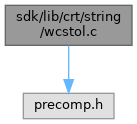ReactOS  0.4.15-dev-3187-ge372f2b
wcstol.c File Reference
`#include <precomp.h>`
Include dependency graph for wcstol.c:Go to the source code of this file.

## Functions

long CDECL wcstol (const wchar_t *nptr, wchar_t **endptr, int base)

## ◆ wcstol()

 long CDECL wcstol ( const wchar_t * nptr, wchar_t ** endptr, int base )

Definition at line 17 of file wcstol.c.

18 {
19  const wchar_t *s = nptr;
20  long acc;
21  int c;
22  unsigned long cutoff;
23  int neg = 0, any, cutlim;
24
25  /*
26  * Skip white space and pick up leading +/- sign if any.
27  * If base is 0, allow 0x for hex and 0 for octal, else
28  * assume decimal; if base is already 16, allow 0x.
29  */
30  do {
31  c = *s++;
32  } while (iswctype(c, _SPACE));
33  if (c == '-')
34  {
35  neg = 1;
36  c = *s++;
37  }
38  else if (c == L'+')
39  c = *s++;
40  if ((base == 0 || base == 16) &&
41  c == L'0' && (*s == L'x' || *s == L'X'))
42  {
43  c = s;
44  s += 2;
45  base = 16;
46  }
47  if (base == 0)
48  base = c == L'0' ? 8 : 10;
49
50  /*
51  * Compute the cutoff value between legal numbers and illegal
52  * numbers. That is the largest legal value, divided by the
53  * base. An input number that is greater than this value, if
54  * followed by a legal input character, is too big. One that
55  * is equal to this value may be valid or not; the limit
56  * between valid and invalid numbers is then based on the last
57  * digit. For instance, if the range for longs is
58  * [-2147483648..2147483647] and the input base is 10,
59  * cutoff will be set to 214748364 and cutlim to either
60  * 7 (neg==0) or 8 (neg==1), meaning that if we have accumulated
61  * a value > 214748364, or equal but the next digit is > 7 (or 8),
62  * the number is too big, and we will return a range error.
63  *
64  * Set any if any `digits' consumed; make it negative to indicate
65  * overflow.
66  */
67  cutoff = neg ? ((unsigned long)LONG_MAX+1) : LONG_MAX;
68  cutlim = cutoff % (unsigned long)base;
69  cutoff /= (unsigned long)base;
70  for (acc = 0, any = 0;; c = *s++)
71  {
72  if (iswctype(c, _DIGIT))
73  c -= L'0';
74  else if (iswctype(c, _ALPHA))
75  c -= iswctype(c, _UPPER) ? L'A' - 10 : L'a' - 10;
76  else
77  break;
78  if (c >= base)
79  break;
80  if (any < 0 || (unsigned long)acc > cutoff || (acc == cutoff && c > cutlim))
81  any = -1;
82  else
83  {
84  any = 1;
85  acc *= base;
86  acc += c;
87  }
88  }
89  if (any < 0)
90  {
91  acc = neg ? LONG_MIN : LONG_MAX;
92  }
93  else if (neg)
94  acc = 0-acc;
95  if (endptr != 0)
96  *endptr = any ? (wchar_t *)((size_t)(s - 1)) : (wchar_t *)((size_t)nptr);
97  return acc;
98 }
#define _UPPER
Definition: ctype.h:65
GLuint base
Definition: 3dtext.c:35
#define _SPACE
Definition: ctype.h:68
unsigned short wchar_t
Definition: crtdefs.h:345
__kernel_size_t size_t
Definition: linux.h:237
const GLubyte * c
Definition: glext.h:8905
int __cdecl iswctype(wint_t wc, wctype_t wctypeFlags)
Definition: freeldr.c:97
#define LONG_MAX
Definition: limits.h:43
static const WCHAR L[]
Definition: oid.c:1250
GLdouble s
Definition: gl.h:2039
#define _DIGIT
Definition: ctype.h:67
#define long
Definition: qsort.c:33
#define c
Definition: ke_i.h:80
#define _ALPHA
Definition: ctype.h:76
#define LONG_MIN
Definition: limits.h:42